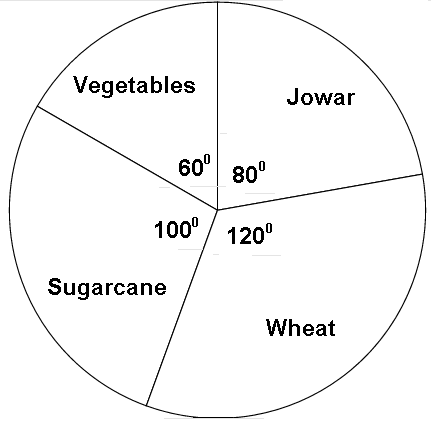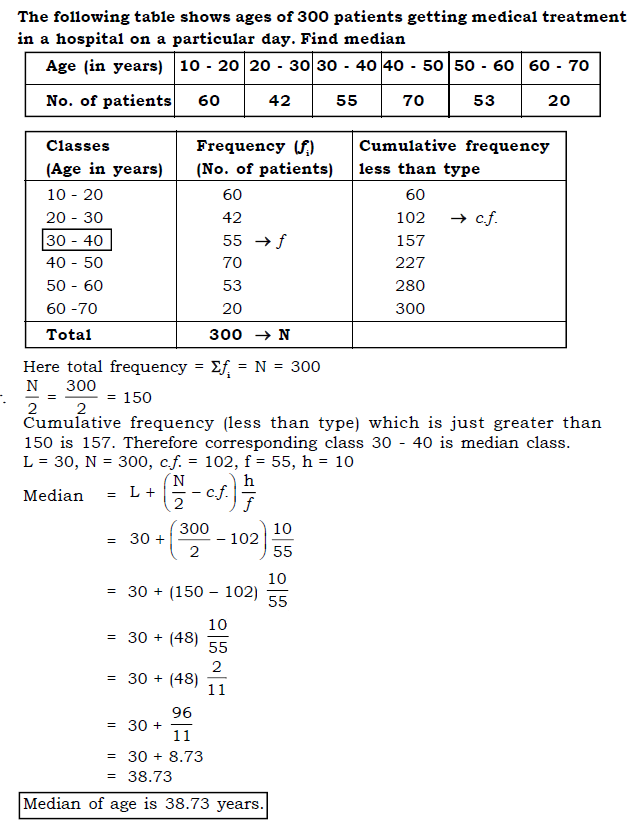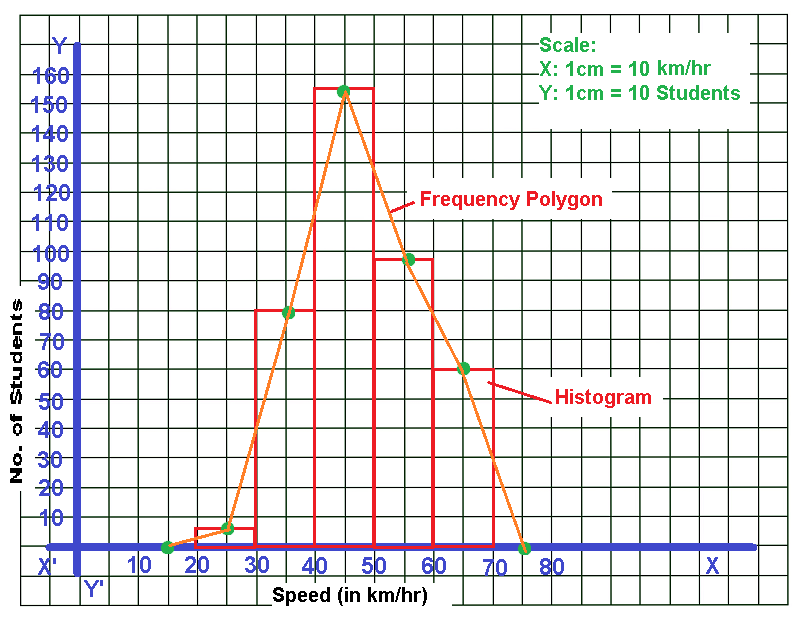PRINTABLE FOR KIDS

XII (12) HSC

XI (11) FYJC
X (10) SSC

### Algebra March 2015 Board Paper with Solution

 Q.P. SET CODE SEAT NO. A N 217 – w ALGEBRA PAPER – I NEW COURSE 2015 MATHEMATICS (71) MAX MARKS: 40 (E) BOARD PAPER
Note : - (i) All question are compulsory.
(ii) Use of calculator is not allowed.
Solution of Algebra March 2015 Board Paper

1. Attempt any five of the following subquestions: 

(i) State whether the following sequence is an A.P. or not? [VIDEO]

1, 4, 7, 10, …..

Ans. Here t1 = 1, t2 = 4, t3 = 7, t4 = 10.

∴ t2 - t1 = 4 - 1 = 3

∴ t3 - t2 = 7 - 4 = 3

∴ t4 - t3 = 10 - 7 = 3

∴ The common difference between any two consecutive terms of  the sequence is same.

∴ It is an A.P.

(ii) A card is drawn from the pack of 25 cards labelled with numbers 1 to 25. Write the sample space for this random experiment. [VIDEO]

Ans. Since a card is drawn from a pack of 25 cards labelled with numbers 1 to 25.

∴ Sample Space,

S = { 1, 2, 3, …, 25}

∴ n(S) = 25

(iii) Find the value of x + y ,  if [VIDEO]

12x + 13y = 29 and
13x + 12y = 21.

Ans: 12x + 13y = 29 _________________ equation no. (1)
13x + 12y = 21 _____________________ equation no. (2)

Adding equation no. (1) & (2)
12x + 13y = 29
13x + 12y = 21
____________
25x + 25y = 50
____________

∴ 25 (x + y) = 50

∴ x + y = 50 ÷ 25

∴ x + y = 2

(iv) For a sequence if [Video]
Sn = n ÷ (n+1)

then find the value of S10.

Ans. Sn = n ÷ (n+1) [Given]

∴  S10 = 10 ÷ (10 + 1)

∴  S10 = 10/11

(v) Verify whether 1 is the root of the quadratic equation : [VIDEO]

x2 + 3x - 4 = 0.

Ans. Given quadratic equation is x2 + 3x - 4 = 0.

If 1 is the root of the quadratic equation then it will satisfy the given quadratic equation.

∴ Let us check by substituting, whether 1 satisfies the equation or not.

Put  x = 1 in given equation x2 + 3x - 4 = 0

∴ 12 + 3(1) - 4 = 0

∴ 1 + 3 - 4 = 0

∴ 4 - 4 = 0

∴ 0 = 0.

Here, the LHS and RHS are equal.

Therefore 1 is the root of the given quadratic equation x2 + 3x - 4 = 0

(vi) If x + y = 5  and  x = 3,   then find the value of y. [VIDEO]

Ans. x + y = 5 [Given]
The value of one unknown variable x = 3 [Given]

∴ 3 + y = 5

∴ y = 5 - 3

∴ y = 2

2. Attempt any four of the following subquestions: 

1. Solve the following quadratic equation by factorization method. [VIDEO]
x2 - 7x + 12 = 0 .

Ans. The given quadratic equation is x2 - 7x + 12 = 0

∴ x2 - 4x - 3x + 12 = 0

∴ x(x - 4) - 3 (x - 4) = 0

∴ (x - 3) (x - 4) = 0

∴ (x - 3) = 0   OR   (x - 4) = 0

∴ x - 3 = 0  OR x - 4 = 0

∴ x = 3  OR x = 4.

2. Find the term t10 of an A.P. :
4, 9, 14, …….

Ans. The given sequence is an A.P. with

a = 4 and

d = t2 - t1

∴ d = 9 - 4

∴ d = 5

We Know That, tn = a + (n - 1)d

∴ t10 = 4 + (10 - 1) 5

∴ t10 = 4 + (9) 5

∴ t10 = 4 + 45

∴ t10 = 49.

3. If the point A(2, 3) lies on the graph of the equation 5x + ay = 19, then find a. [VIDEO]

Ans. Since the Point A (2, 3) lies on the graph of the equation 5x + ay = 19.

∴ It satisfies the given linear equation.

Now, we substitute x = 2 and y = 3 in the given equation

∴ 5(2) + a(3) = 19

∴ 10 + 3a = 19

∴ 3a = 19 - 10

∴ 3a = 9

∴ a = 9/3

∴ a = 3.

4. A die is thrown. If A is an event of getting an odd number, then write the sample space and event A  in set notation. [VIDEO]

Ans. Since a die is thrown,

∴ Sample Space, S = {1, 2, 3, 4, 5, 6 }

∴ n(S) = 6

A = Event of getting an odd number. [Given]

∴ A = {1, 3, 5}

∴ n(A) = 3.

5. For a certain frequency distribution, the value of Mean is 101 and Median is 100. Find the value of Mode. [VIDEO]

Ans. Here, Mean = 101  and Median = 100.

Mean = 101, Median = 100 [Given]
We know that,
Mean – Mode = 3 (Mean – Median)
∴  101 – Mode = 3 (101 – 100)
∴  101 – Mode = 3 (1)
∴  101 – 3 = Mode

∴  Mode = 98

6. If one root of the quadratic equation [VIDEO]
kx2 - 7x + 5 = 0
is 1, then find the value of k.

Ans. Since 1 the root of the quadratic equation kx2 - 7x + 5 = 0

∴ it will satisfy the given quadratic equation

∴ Substituting x = 1 in given quadratic equation

∴ kx2 - 7x + 5 = 0

∴ k(1)2 - 7(1) + 5 = 0

∴ k - 7 + 5 = 0

∴ k - 2 = 0

∴ k = 2.

3. Attempt any three of the following sub questions:

1. Area under different crops in a certain village is given below. Represent it by a pie diagram. [VIDEO]

 Crop Area in Hectares Jowar 40 Wheat 60 Sugarcane 50 Vegetables 30

Ans.

 Crop Area in Hectares Measure of Central Angle (Θ) Jowar 40 (40 ÷ 180) × 360 = 800 Wheat 60 (60 ÷ 180) × 360 = 1200 Sugarcane 50 (50 ÷ 180) × 360 = 1000 Vegetables 30 (30 ÷ 180) × 360 = 600 Total 180 36002. If two coins are tossed, then find the probability of the event that at the most one tail turns up. [VIDEO]

Ans. Since two coins are tossed,

∴ The Sample Space is,

S = { HH, HT, TH, TT}

∴ n(S) = 4

Let A = Event of getting at the most one tail turns up in a toss.

∴ A = {HH, HT, TH}

∴ n(A) = 3

∴ P(A) = n(A) ÷ n(S)

∴ P(A) = 3 ÷ 4

∴ P(A) = ¾

3. Solve the following simultaneous equations using graphical method. [VIDEO]
x + y = 7;
x - y = 5.

Ans. x + y = 7 [Given]

Let us substitute the value of x as 0, 1 and 2 in the given simultaneous equation.
We will get the value of y as 7, 6 and 5 respectively.

 x 0 1 2 y 7 6 5 ( x , y ) ( 0, 7 ) ( 1, 6 ) ( 2, 5 )

Now, we take the second equation x - y = 5,

Here we substitute the value of y as 0, 1 and 2 then we will get the value of x as 5, 6 and 7 respectively.

 x 5 6 7 y 0 1 2 ( x , y ) ( 5, 0 ) (6, 1) (7, 2)4. There is an auditorium with 35 rows of seats. There are 20 seats in the first row, 22 seats in the second row, 24 seats in the third row and so on. Find the number of seats in the twenty second row. [VIDEO]

Ans. Since the no. of seats in each row of the  auditorium are 20, 22, 24, ......
The no. of seats in each row form an A.P.

No. of seats in first row (a) = 20

Difference in no. of seats in two successive rows is (d) = 2

No. of seats in 22nd row = t22 = ?

tn = a + (n + 1) d

t22 = a + (22 – 1) d
t22 = 20 + 21 (2)
t22 = 20 + 42
t22 = 62
There are 62 seats in 22th row.

5. Solve the following quadratic equation by completing square method.
x2 + 11x + 24 = 0. [VIDEO]
Ans. The Given Equation is x2 + 11x + 24 = 0

∴ x2 + 11x = - 24 ------------------------- eq (1)

Third Term = [½ × Coefficient of x]2

∴ Third Term = [½ × 11]2

∴ Third Term = [11/2]2

∴ Third Term = 121/4

Adding Third term on both the sides in equation no. (1)

∴ x2  + 11x + 121/4 = - 24 + 121/4

∴ (x + 11/2)2 = (-96+121)/4

∴ (x + 11/2)2 = 25/4

Taking Square root on both the sides, after that we will get,

∴ x + 11/2 = ± 5/2

∴ x = - 11/2 ± 5/2

∴ x = -11 ± 52

∴ x = -11 + 52 or -11 - 52

∴ x = - 6 2  or  -162

∴ x = - 3  or  - 8

4. Attempt any two of the following subquestions: 

1. Two digit number are formed using the digits 0, 1, 2, 3, 4, 5, where digits are not repeated.
P is the event that the number so formed is even.
Q is the event that the number so formed is greater than 50.
R is the event that the number so formed is divisible by 3.
Then write the sample space S and events P, Q, R using set notation.

Ans. The two digit numbers that can be formed using the digits 0, 1, 2, 3, 4, 5 without repeating digits are as follows :

S = { 10, 12, 13, 14, 15, 20, 21, 23, 24, 25, 30, 31, 32, 34, 35, 40, 41, 42, 43, 45, 50, 51, 52, 53, 54 }
n (S) = 25
P is the event that number so formed is even
P = { 10, 12, 14, 20, 24, 30, 32, 34, 40, 42, 50, 52, 54 }
∴  n (P) = 13

Q is the event that the number so formed is greater than 50
Q = { 51, 52, 53, 54 }

∴  n (Q) = 4

R is the event that number so formed is divisible by 3
R = { 12, 15, 21, 24, 30, 42, 45, 51, 54 }
∴  n (R) = 9

2. The following table shows ages of 300 patients getting medical treatment in a hospital on a particular day.

 Age ( in years) No. of Patients. 10 - 20 60 20 - 30 42 30 - 40 55 40 - 50 70 50 - 60 53 60 - 70 20

Find the median age of the patients.

Ans.3. If α + β = 5 and α3 + β3 = 35, find the quadratic equation whose roots are α and β.

Ans. Let α  and β  be the roots of a quadratic equation.

∴  α  + β  = 5 [Given] ...... eq. (1)

and α3 + β3 = 35  ............ eq. (2)

We know that,

α3 + β3 = (α + β )3 – 3αβ (α + β )

∴ 35 = 53 – 3αβ (5)   [ From eq. (1) & (2)]

∴ 35 – 125 = - 15αβ

∴ -90 = - 15αβ

∴ α β = - 90/-15

∴ α β = 6

We know that, Quadratic equation is given by,

x2 – (Sum of the roots)x + Product of the roots = 0

∴ x2 –( α  + β)x + αβ = 0

∴ x2 – 5x + 6 = 0  is the required quadratic equation.

5. Attempt any two of the following subquestions: 

1. Babubhai borrows Rs. 4000 and agrees to repay with a total interest of Rs, 500 in 10 instalments, each instalment being less than the preceding instalment by Rs. 10. What should be the first and the last instalments?

Ans. Total money repaid by Babubhai in 10 instalments = (S10)= Principle + Interest
S10 = 4000 + 500
S10 = Rs. 4500

No. of instalments (n) = 10

He repays each instalment being less than the preceding instalment by Rs. 10.

Difference between two consecutive instalments (d) = – 10

First instalment = (a) = ?

Last instalment (t10) = ?

We know that,
Sn = n/2[2a + (n – 1) d]
S10 = 10/2 [2a + (10 – 1) d]
4500 = 5 [2a + 9 (– 10)]
4500/5 = 2a – 90
900 = 2a – 90
900 + 90 = 2a
990 = 2a
a = 990/2
a = 495 ............. eq. (i)
Now, tn = a + (n – 1) d
t10 = a + (10 – 1) d
t10 = 495 + 9 (– 10) [From eq. (i)]
t10 = 495 – 90
t10 = 405

First instalment is Rs. 495 and last instalment is Rs.405

2. On the first day of the sale of tickets of a drama, in all 35 tickets were sold. If the rates of the tickets were Rs.  20 and Rs. 40. per ticket and the total collection was Rs. 900, find the number of tickets sold of each rate.

Ans. Let the number of tickets sold at the rate of Rs. 20 be x and at the rate of  Rs. 40 be y.

According to first condition,

No. of tickets sold @ Rs. 20 + No. of tickets sold @ Rs. 40 = Total No. of Tickets sold (i.e.) 35 tickets

∴ x + y = 35 _____________ eq. (1)

According to second condition,

Amount received by selling Rs. 20 tickets + Amount Received by selling Rs. 40 Tickets = Total Collection received (i.e.) Rs. 900

∴ 20x +40y = 900

∴ 20(x + 2y) = 900

∴ x + 2y = 900/20

∴ x + 2y = 45 ________________ eq. no. (2)

Subtracting Equation (1) From Equation (2)

x + 2y = 45
x +   y = 35
(-)   (-)   (-)
__________
y =   10
_________

Substituting y = 15 in equation (1)

∴ x + y = 35

∴ x + 10 = 35

∴ x = 35 - 10

∴ x = 25

∴ 25 no. of Rs. 20 Tickets and 10 no. of Rs. 40 Tickets were sold on the first day of the sale of tickets of a drama.

3. Given below is the frequency distribution of driving speeds (in km/hour) of the vehicles of 400 college students:

 Speed ( in km/hr) No. of Students 20 - 30 6 30 - 40 80 40 - 50 156 50 - 60 98 60 - 70 60
Draw Histogram and hence the frequency polygon for the above data.

Ans.## PDF FILE TO YOUR EMAIL IMMEDIATELY PURCHASE NOTES & PAPER SOLUTION. @ Rs. 50/- each (GST extra)

SUBJECTS

HINDI ENTIRE PAPER SOLUTION

MARATHI PAPER SOLUTION
SSC MATHS I PAPER SOLUTION
SSC MATHS II PAPER SOLUTION
SSC SCIENCE I PAPER SOLUTION
SSC SCIENCE II PAPER SOLUTION
SSC ENGLISH PAPER SOLUTION
SSC & HSC ENGLISH WRITING SKILL
HSC ACCOUNTS NOTES
HSC OCM NOTES
HSC ECONOMICS NOTES
HSC SECRETARIAL PRACTICE NOTES

2019 Board Paper Solution

HSC ENGLISH SET A 2019 21st February, 2019

HSC ENGLISH SET B 2019 21st February, 2019

HSC ENGLISH SET C 2019 21st February, 2019

HSC ENGLISH SET D 2019 21st February, 2019

SECRETARIAL PRACTICE (S.P) 2019 25th February, 2019

HSC XII PHYSICS 2019 25th February, 2019

CHEMISTRY XII HSC SOLUTION 27th, February, 2019

OCM PAPER SOLUTION 2019 27th, February, 2019

HSC MATHS PAPER SOLUTION COMMERCE, 2nd March, 2019

HSC MATHS PAPER SOLUTION SCIENCE 2nd, March, 2019

SSC ENGLISH STD 10 5TH MARCH, 2019.

HSC XII ACCOUNTS 2019 6th March, 2019

HSC XII BIOLOGY 2019 6TH March, 2019

HSC XII ECONOMICS 9Th March 2019

SSC Maths I March 2019 Solution 10th Standard11th, March, 2019

SSC MATHS II MARCH 2019 SOLUTION 10TH STD.13th March, 2019

SSC SCIENCE I MARCH 2019 SOLUTION 10TH STD. 15th March, 2019.

SSC SCIENCE II MARCH 2019 SOLUTION 10TH STD. 18th March, 2019.

SSC SOCIAL SCIENCE I MARCH 2019 SOLUTION20th March, 2019

SSC SOCIAL SCIENCE II MARCH 2019 SOLUTION, 22nd March, 2019

XII CBSE - BOARD - MARCH - 2019 ENGLISH - QP + SOLUTIONS, 2nd March, 2019

HSC Maharashtra Board Papers 2020

(Std 12th English Medium)

HSC ECONOMICS MARCH 2020

HSC OCM MARCH 2020

HSC ACCOUNTS MARCH 2020

HSC S.P. MARCH 2020

HSC ENGLISH MARCH 2020

HSC HINDI MARCH 2020

HSC MARATHI MARCH 2020

HSC MATHS MARCH 2020

SSC Maharashtra Board Papers 2020

(Std 10th English Medium)

English MARCH 2020

HindI MARCH 2020

Hindi (Composite) MARCH 2020

Marathi MARCH 2020

Mathematics (Paper 1) MARCH 2020

Mathematics (Paper 2) MARCH 2020

Sanskrit MARCH 2020

Important-formula

THANKS